1646961240

# Dexplot: Wraps Matplotlib and Integrated with DataFrames

## Dexplot

Dexplot is a Python library for delivering beautiful data visualizations with a simple and intuitive user experience.

## Goals

The primary goals for dexplot are:

• Maintain a very consistent API with as few functions as necessary to make the desired statistical plots
• Allow the user tremendous power without using matplotlib

## Installation

`pip install dexplot`

## Built for long and wide data

Dexplot is primarily built for long data, which is a form of data where each row represents a single observation and each column represents a distinct quantity. It is often referred to as "tidy" data. Here, we have some long data.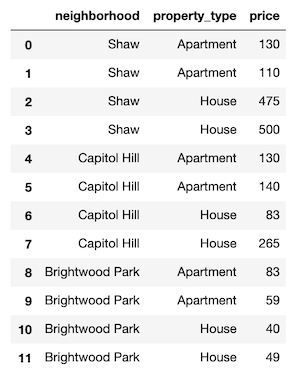Dexplot also has the ability to handle wide data, where multiple columns may contain values that represent the same kind of quantity. The same data above has been aggregated to show the mean for each combination of neighborhood and property type. It is now wide data as each column contains the same quantity (price).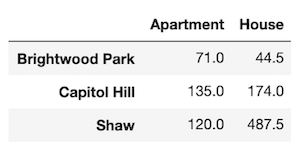## Usage

Dexplot provides a small number of powerful functions that all work similarly. Most plotting functions have the following signature:

``````dxp.plotting_func(x, y, data, aggfunc, split, row, col, orientation, ...)
``````
• `x` - Column name along the x-axis
• `y` - Column name the y-axis
• `data` - Pandas DataFrame
• `aggfunc` - String of pandas aggregation function, 'min', 'max', 'mean', etc...
• `split` - Column name to split data into distinct groups
• `row` - Column name to split data into distinct subplots row-wise
• `col` - Column name to split data into distinct subplots column-wise
• `orientation` - Either vertical (`'v'`) or horizontal (`'h'`). Default for most plots is vertical.

When `aggfunc` is provided, `x` will be the grouping variable and `y` will be aggregated when vertical and vice-versa when horizontal. The best way to learn how to use dexplot is with the examples below.

## Families of plots

There are two primary families of plots, aggregation and distribution. Aggregation plots take a sequence of values and return a single value using the function provided to `aggfunc` to do so. Distribution plots take a sequence of values and depict the shape of the distribution in some manner.

• Aggregation
• bar
• line
• scatter
• count
• Distribution
• box
• violin
• hist
• kde

## Comparison with Seaborn

If you have used the seaborn library, then you should notice a lot of similarities. Much of dexplot was inspired by Seaborn. Below is a list of the extra features in dexplot not found in seaborn

• Ability to graph relative frequency and normalize over any number of variables
• No need for multiple functions to do the same thing (far fewer public functions)
• Ability to make grids with a single function instead of having to use a higher level function like `catplot`
• Pandas `groupby` methods available as strings
• Ability to sort by values
• Ability to sort x/y labels lexicographically
• Ability to select most/least frequent groups
• x/y labels are wrapped so that they don't overlap
• Figure size (plus several other options) and available to change without using matplotlib
• A matplotlib figure object is returned

## Examples

Most of the examples below use long data.

## Aggregating plots - bar, line and scatter

We'll begin by covering the plots that aggregate. An aggregation is defined as a function that summarizes a sequence of numbers with a single value. The examples come from the Airbnb dataset, which contains many property rental listings from the Washington D.C. area.

``````import dexplot as dxp
import pandas as pd
``````

There are more than 4,000 listings in our dataset. We will use bar charts to aggregate the data.

``````airbnb.shape
``````
``````(4581, 12)
``````

### Vertical bar charts

In order to performa an aggregation, you must supply a value for `aggfunc`. Here, we find the median price per neighborhood. Notice that the column names automatically wrap.

``````dxp.bar(x='neighborhood', y='price', data=airbnb, aggfunc='median')
``````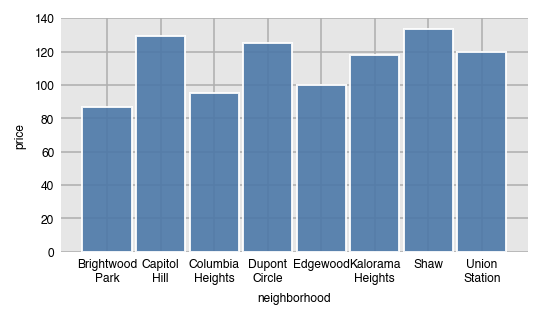Line and scatter plots can be created with the same command, just substituting the name of the function. They both are not good choices for the visualization since the grouping variable (neighborhood) has no meaningful order.

``````dxp.line(x='neighborhood', y='price', data=airbnb, aggfunc='median')
``````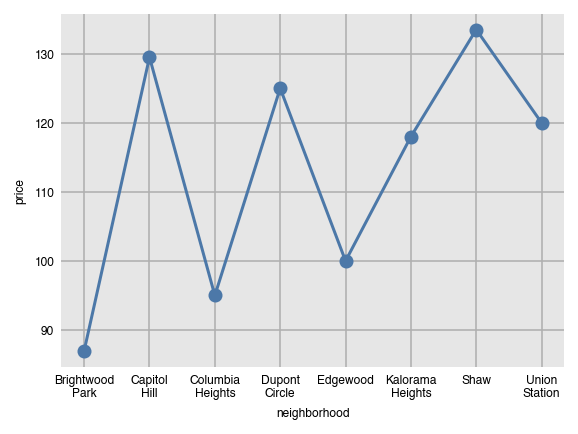``````dxp.scatter(x='neighborhood', y='price', data=airbnb, aggfunc='median')
``````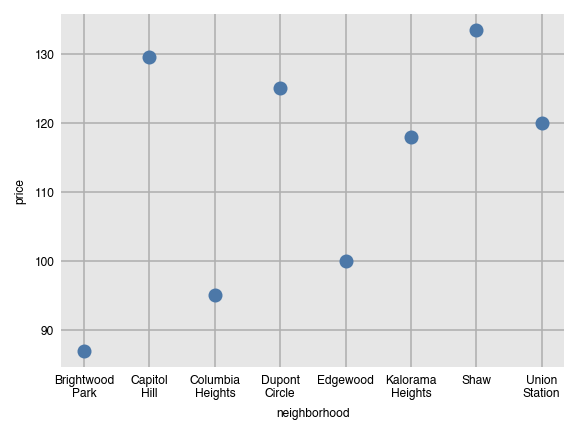### Components of the groupby aggregation

Anytime the `aggfunc` parameter is set, you have performed a groupby aggregation, which always consists of three components:

• Grouping column - unique values of this column form independent groups (neighborhood)
• Aggregating column - the column that will get summarized with a single value (price)
• Aggregating function - a function that returns a single value (median)

The general format for doing this in pandas is:

``````df.groupby('grouping column').agg({'aggregating column': 'aggregating function'})
``````

Specifically, the following code is executed within dexplot.

``````airbnb.groupby('neighborhood').agg({'price': 'median'})
``````

### Number and percent of missing values with `'countna'` and `'percna'`

In addition to all the common aggregating functions, you can use the strings `'countna'` and `'percna'` to get the number and percentage of missing values per group.

``````dxp.bar(x='neighborhood', y='response_time', data=airbnb, aggfunc='countna')
``````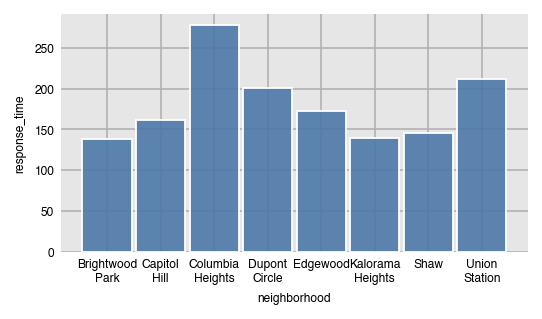### Sorting the bars by values

By default, the bars will be sorted by the grouping column (x-axis here) in alphabetical order. Use the `sort_values` parameter to sort the bars by value.

• None - sort x/y axis labels alphabetically (default)
• `asc` - sort values from least to greatest
• `desc` - sort values from greatest to least
``````dxp.bar(x='neighborhood', y='price', data=airbnb, aggfunc='median', sort_values='asc')
``````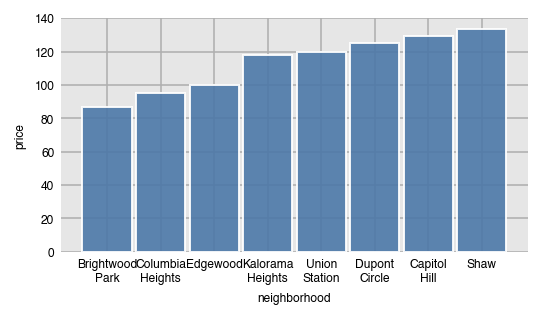Here, we sort the values from greatest to least.

``````dxp.bar(x='neighborhood', y='price', data=airbnb, aggfunc='median', sort_values='desc')
``````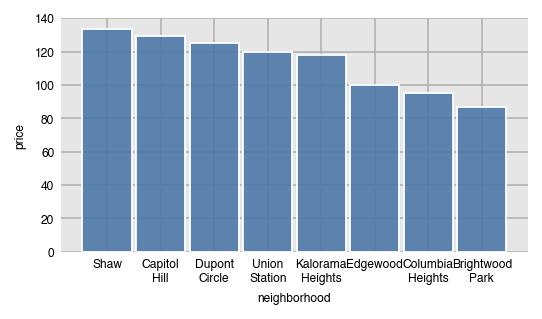### Specify order with `x_order`

Specify a specific order of the labels on the x-axis by passing a list of values to `x_order`. This can also act as a filter to limit the number of bars.

``````dxp.bar(x='neighborhood', y='price', data=airbnb, aggfunc='median',
x_order=['Dupont Circle', 'Edgewood', 'Union Station'])
``````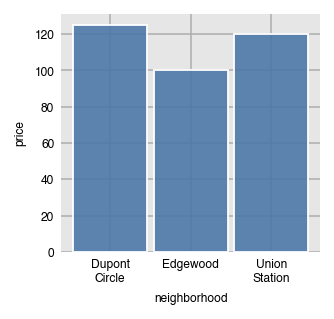By default, `x_order` and all of the `_order` parameters are set to `'asc'` by default, which will order them alphabetically. Use the string `'desc'` to sort in the opposite direction.

``````dxp.bar(x='neighborhood', y='price', data=airbnb, aggfunc='median', x_order='desc')
``````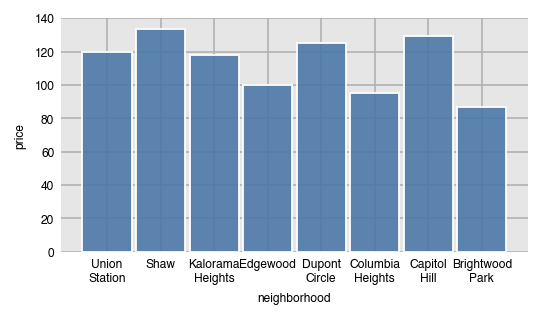### Filter for the neighborhoods with most/least frequency of occurrence

You can use `x_order` again to filter for the x-values that appear the most/least often by setting it to the string `'top n'` or `'bottom n'` where `n` is an integer. Here, we filter for the top 4 most frequently occurring neighborhoods. This option is useful when there are dozens of unique values in the grouping column.

``````dxp.bar(x='neighborhood', y='price', data=airbnb, aggfunc='median',
x_order='top 4')
``````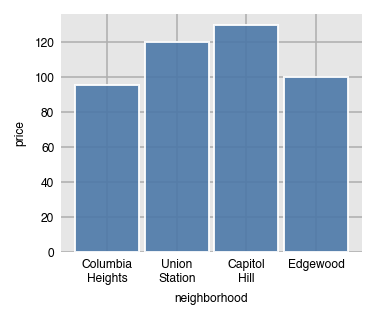We can verify that the four neighborhoods are the most common.

``````airbnb['neighborhood'].value_counts()
``````
``````Columbia Heights    773
Union Station       713
Capitol Hill        654
Edgewood            610
Dupont Circle       549
Shaw                514
Brightwood Park     406
Kalorama Heights    362
Name: neighborhood, dtype: int64
``````

### Horizontal bars

Set `orientation` to `'h'` for horizontal bars. When you do this, you'll need to switch `x` and `y` since the grouping column (neighborhood) will be along the y-axis and the aggregating column (price) will be along the x-axis.

``````dxp.bar(x='price', y='neighborhood', data=airbnb, aggfunc='median',
orientation='h', sort_values='desc')
``````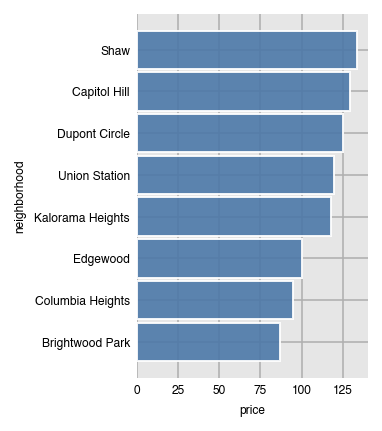Switching orientation is possible for most other plots.

``````dxp.line(x='price', y='neighborhood', data=airbnb, aggfunc='median', orientation='h')
``````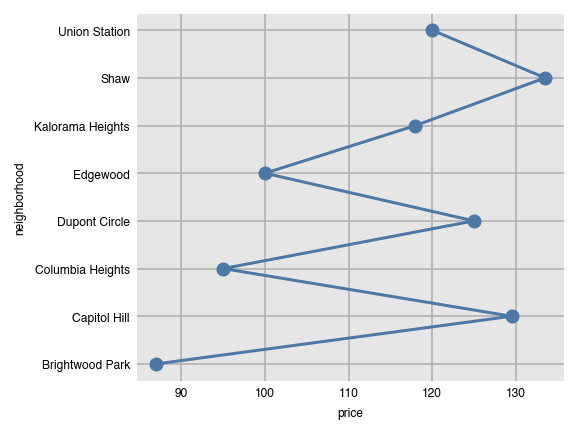### Split bars into groups

You can split each bar into further groups by setting the `split` parameter to another column.

``````dxp.bar(x='neighborhood', y='price', data=airbnb, aggfunc='median', split='superhost')
``````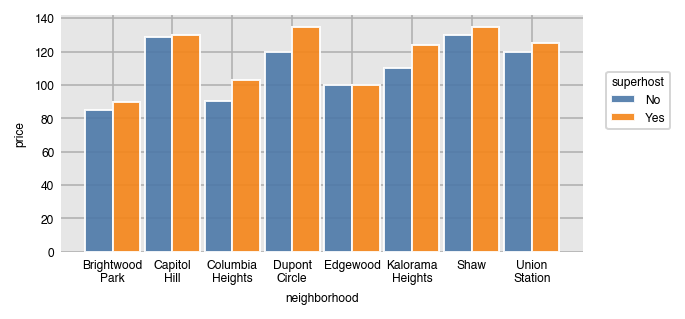We can use the `pivot_table` method to verify the results in pandas.

``````airbnb.pivot_table(index='superhost', columns='neighborhood',
values='price', aggfunc='median')
``````

Set the order of the unique split values with `split_order`, which can also act as a filter.

``````dxp.bar(x='neighborhood', y='price', data=airbnb, aggfunc='median',
split='superhost', split_order=['Yes', 'No'])
``````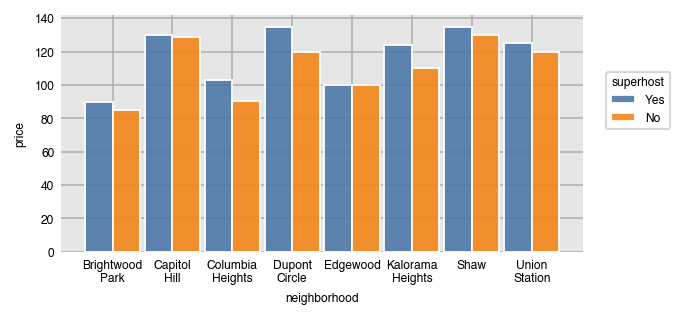Like all the `_order` parameters, `split_order` defaults to `'asc'` (alphabetical) order. Set it to `'desc'` for the opposite.

``````dxp.bar(x='neighborhood', y='price', data=airbnb, aggfunc='median',
split='property_type', split_order='desc')
``````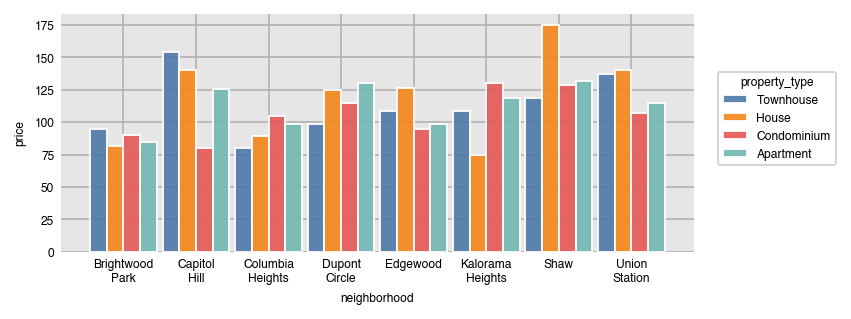Filtering for the most/least frequent split categories is possible.

``````dxp.bar(x='neighborhood', y='price', data=airbnb, aggfunc='median',
split='property_type', split_order='bottom 2')
``````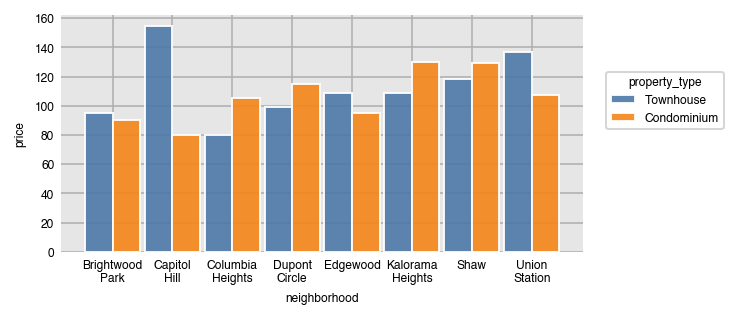Verifying that the least frequent property types are Townhouse and Condominium.

``````airbnb['property_type'].value_counts()
``````
``````Apartment      2403
House           877
Townhouse       824
Condominium     477
Name: property_type, dtype: int64
``````

### Stacked bar charts

Stack all the split groups one on top of the other by setting `stacked` to `True`.

``````dxp.bar(x='neighborhood', y='price', data=airbnb, aggfunc='median',
split='superhost', split_order=['Yes', 'No'], stacked=True)
``````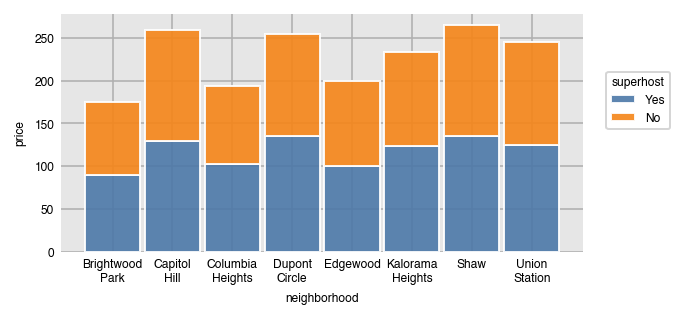### Split into multiple plots

It's possible to split the data further into separate plots by the unique values in a different column with the `row` and `col` parameters. Here, each kind of `property_type` has its own plot.

``````dxp.bar(x='neighborhood', y='price', data=airbnb, aggfunc='median',
split='superhost', col='property_type')
``````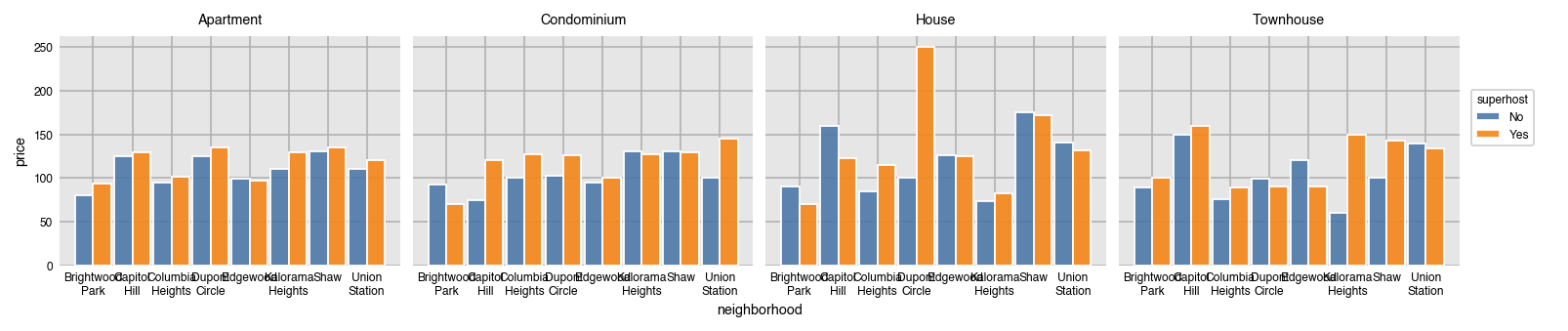If there isn't room for all of the plots, set the `wrap` parameter to an integer to set the maximum number of plots per row/col. We also specify the `col_order` to be descending alphabetically.

``````dxp.bar(x='neighborhood', y='price', data=airbnb, aggfunc='median',
split='superhost', col='property_type', wrap=2, col_order='desc')
``````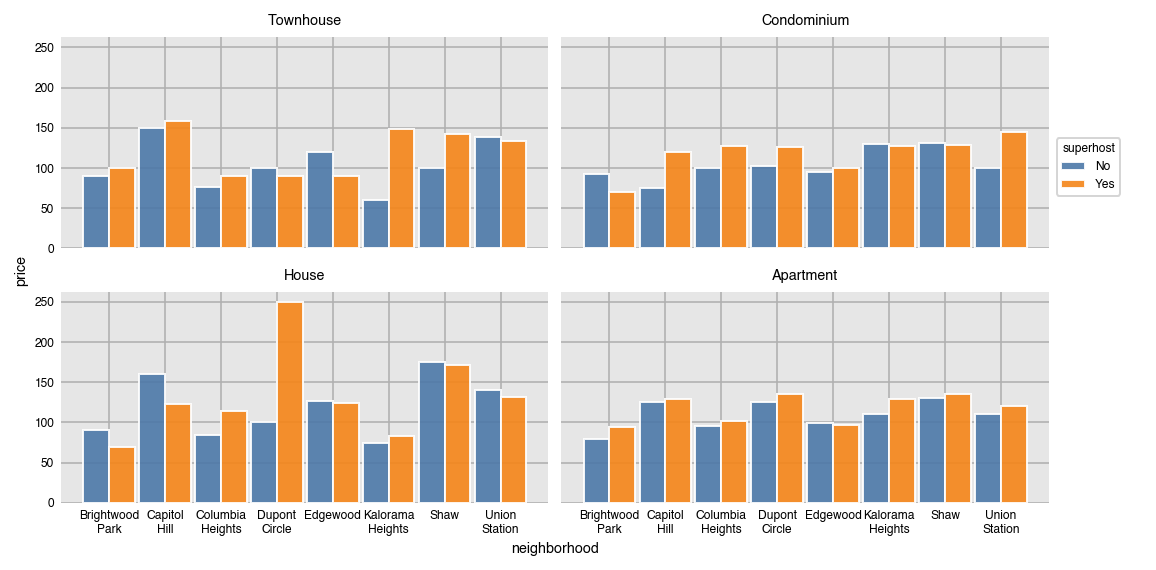Use `col_order` to both filter and set a specific order for the plots.

``````dxp.bar(x='neighborhood', y='price', data=airbnb, aggfunc='median',
split='superhost', col='property_type', col_order=['House', 'Condominium'])
``````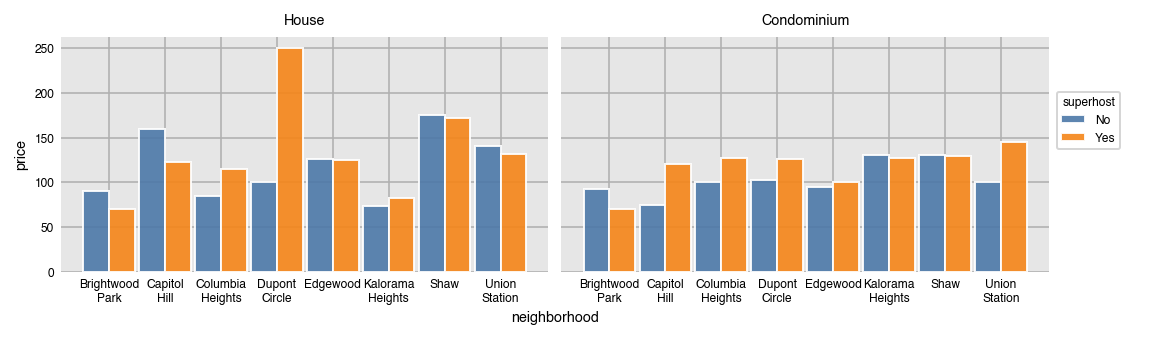Splits can be made simultaneously along row and columns.

``````dxp.bar(x='neighborhood', y='price', data=airbnb, aggfunc='median', split='superhost',
col='property_type', col_order=['House', 'Condominium', 'Apartment'],
row='bedrooms', row_order=[1, 2, 3])
``````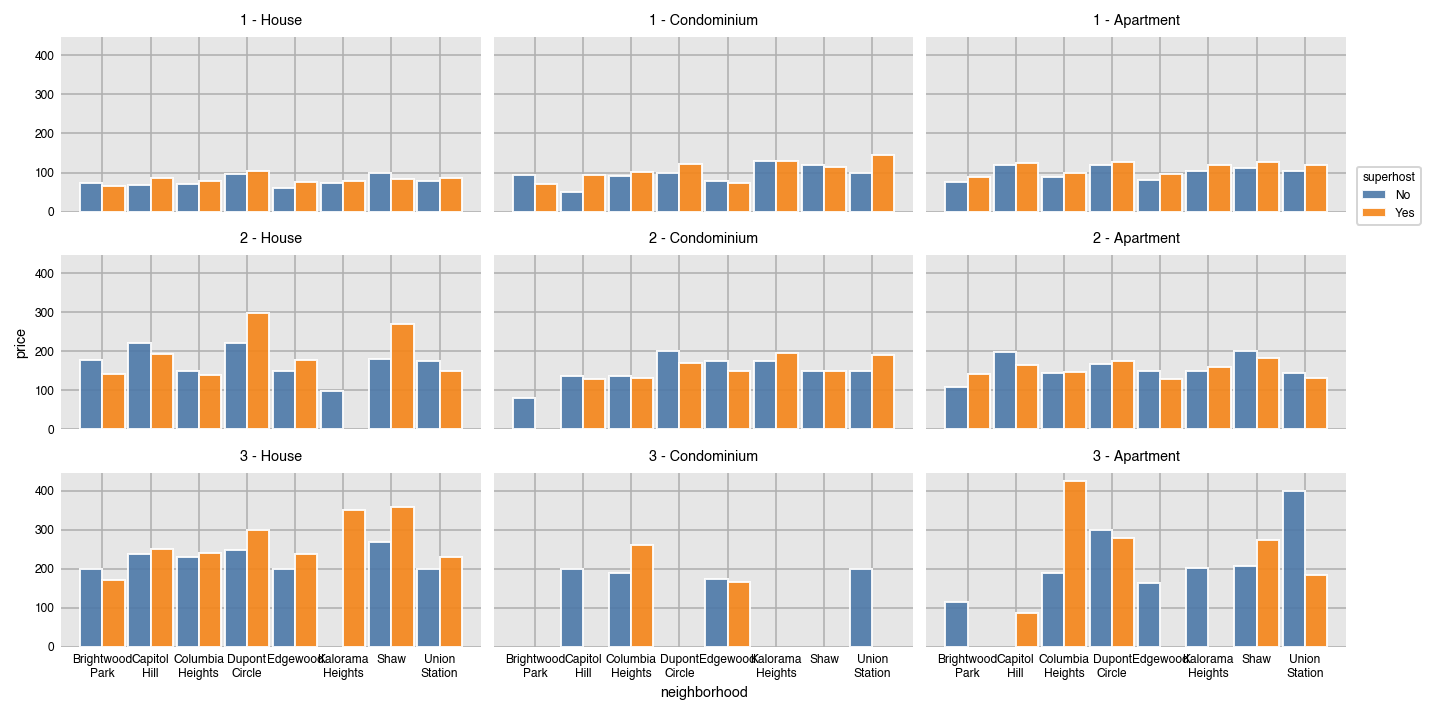By default, all axis limits are shared. Allow each plot to set its own limits by setting `sharex` and `sharey` to `False`.

``````dxp.bar(x='neighborhood', y='price', data=airbnb, aggfunc='median', split='superhost',
col='property_type', col_order=['House', 'Condominium', 'Apartment'],
row='bedrooms', row_order=[1, 2, 3], sharey=False)
``````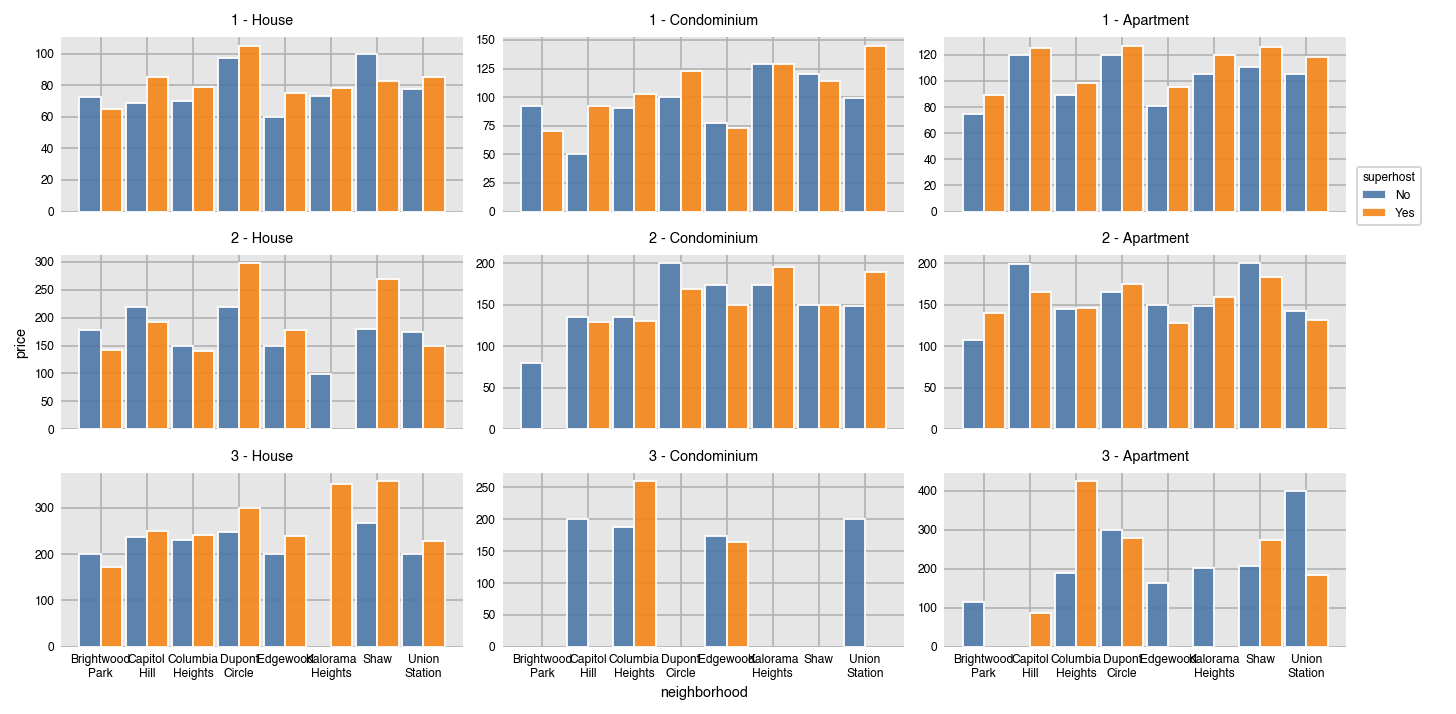### Set the width of each bar with `size`

The width (height when horizontal) of the bars is set with the `size` parameter. By default, this value is .9. Think of this number as the relative width of all the bars for a particular x/y value, where 1 is the distance between each x/y value.

``````dxp.bar(x='neighborhood', y='price', data=airbnb,
aggfunc='median', split='property_type',
split_order=['Apartment', 'House'],
x_order=['Dupont Circle', 'Capitol Hill', 'Union Station'], size=.5)
``````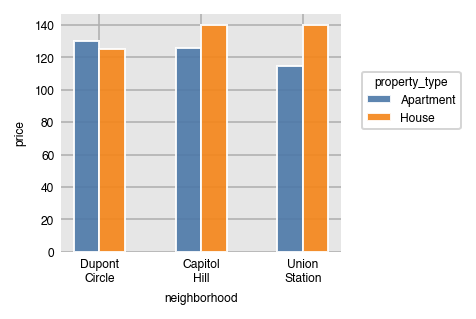### Splitting line plots

All the other aggregating plots work similarly.

``````dxp.line(x='neighborhood', y='price', data=airbnb,
aggfunc='median', split='property_type',
split_order=['Apartment', 'House'],
x_order=['Dupont Circle', 'Capitol Hill', 'Union Station'])
``````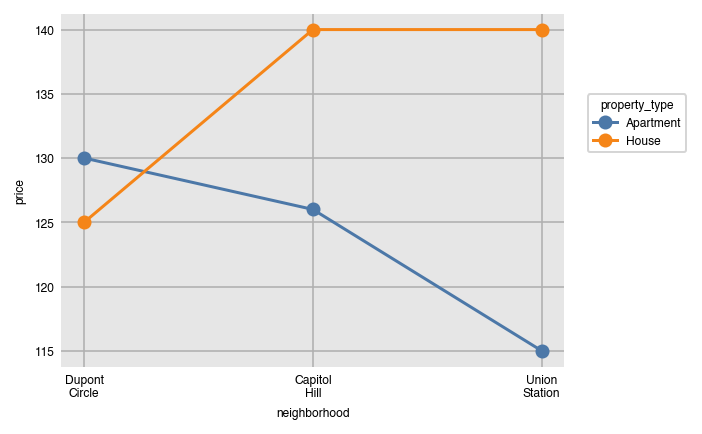## Distribution plots - box, violin, histogram, kde

Distribution plots work similarly, but do not have an `aggfunc` since they do not aggregate. They take their group of values and draw some kind of shape that gives information on how that variable is distributed.

### Box plots

Box plots have colored boxes with ends at the first and third quartiles and a line at the median. The whiskers are placed at 1.5 times the difference between the third and first quartiles (Interquartile range (IQR)). Fliers are the points outside this range and plotted individually. By default, both box and violin plots are plotted horizontally.

``````dxp.box(x='price', y='neighborhood', data=airbnb)
``````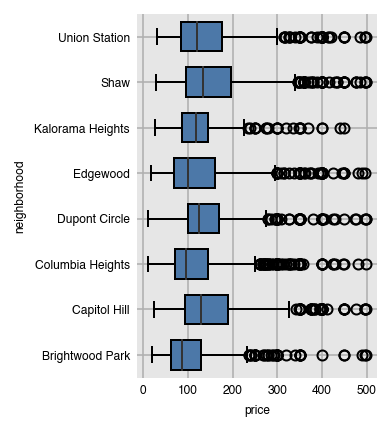Split the groups in the same manner as with the aggregation plots.

``````dxp.box(x='price', y='neighborhood', data=airbnb,
split='superhost', split_order=['Yes', 'No'])
``````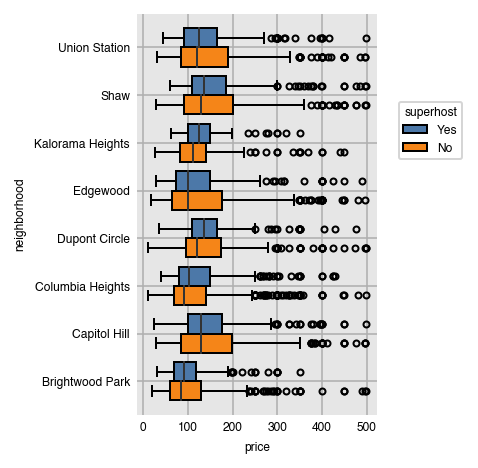Order the appearance of the splits alphabetically (in descending order here).

``````dxp.box(x='price', y='neighborhood', data=airbnb,
split='property_type', split_order='desc')
``````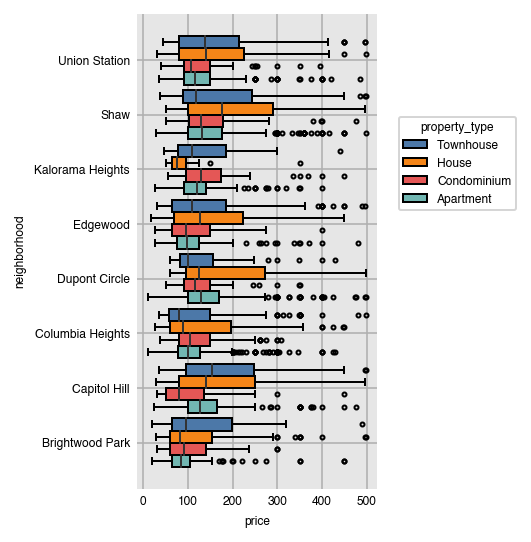### Filter range of values with `x_order`

It's possible to filter the range of possible values by passing in a list of the minimum and maximum to `x_order`.

``````dxp.box(x='price', y='neighborhood', data=airbnb,
split='superhost', x_order=[50, 250])
``````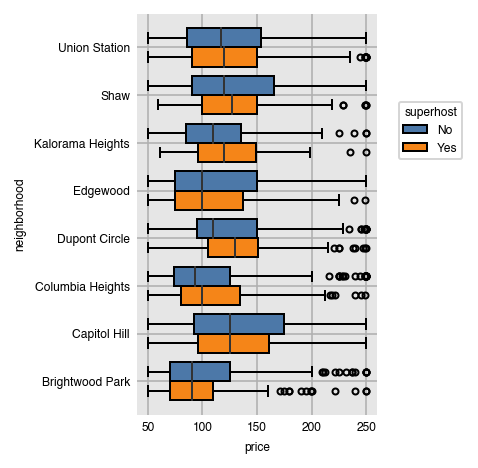Change the `x` and `y` while setting `orientation` to make vertical bar plots.

``````dxp.box(x='neighborhood', y='price', data=airbnb, orientation='v',
split='property_type', split_order='top 2')
``````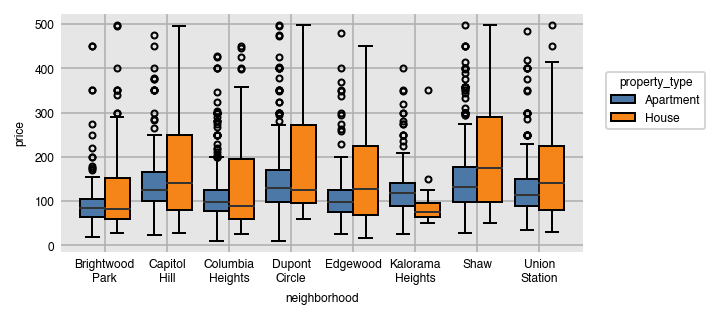Violin plots work identically to box plots, but show "violins", kernel density plots duplicated on both sides of a line.

``````dxp.violin(x='price', y='neighborhood', data=airbnb,
split='superhost', split_order=['Yes', 'No'])
``````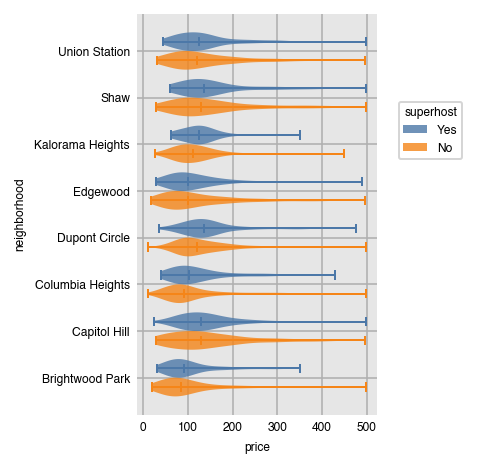Splitting by rows and columns is possible as well with distribution plots.

``````dxp.box(x='price', y='neighborhood', data=airbnb,split='superhost',
col='property_type', col_order=['House', 'Condominium', 'Apartment'],
row='bedrooms', row_order=[1, 2])
``````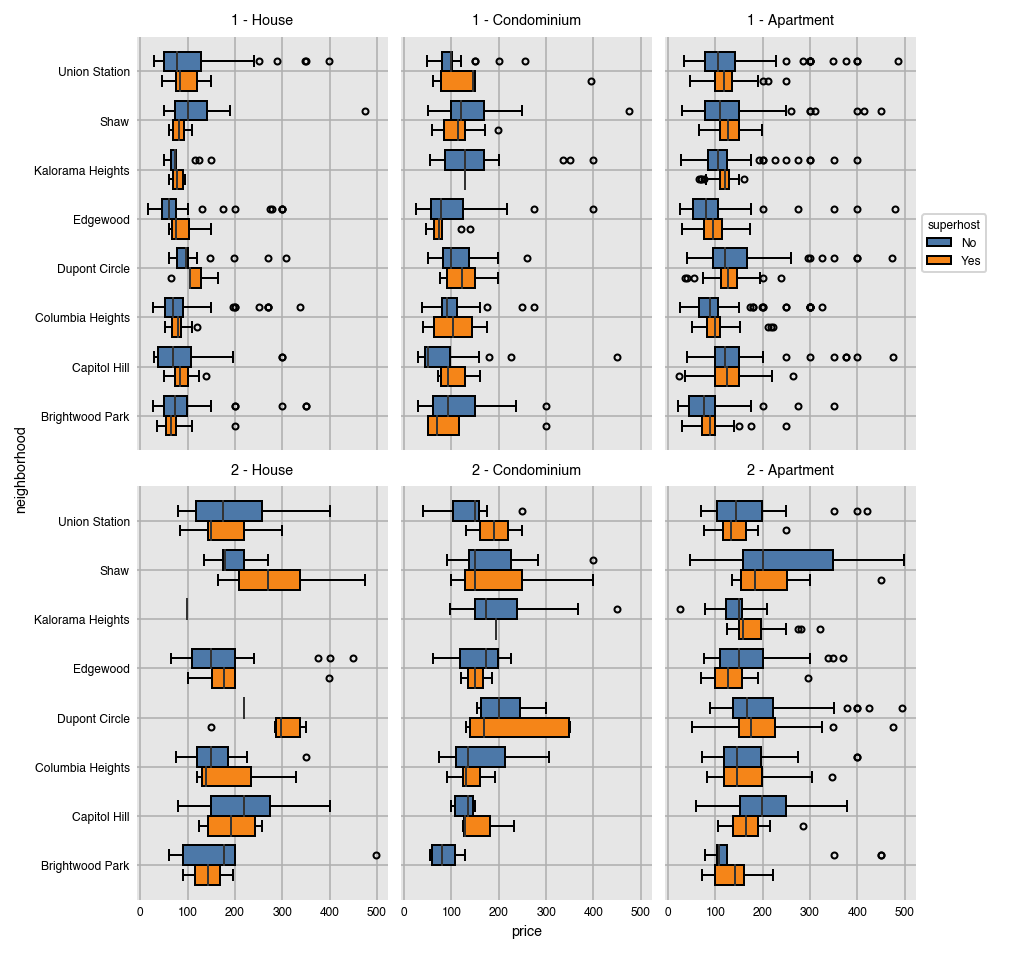### Histograms

Histograms work in a slightly different manner. Instead of passing both `x` and `y`, you give it a single numeric column. A vertical histogram with 20 bins of the counts is created by default.

``````dxp.hist(val='price', data=airbnb)
``````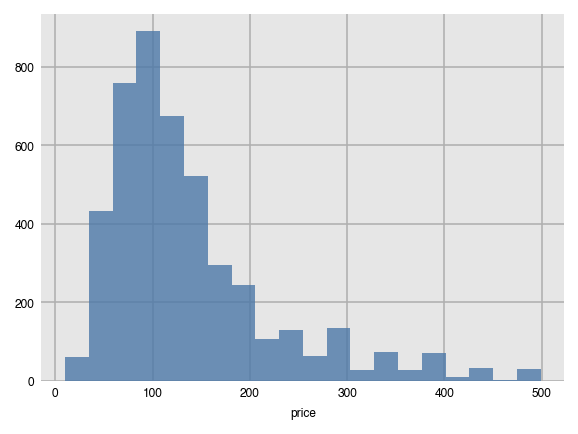We can use `split` just like we did above and also create horizontal histograms.

``````dxp.hist(val='price', data=airbnb, orientation='h', split='superhost', bins=15)
``````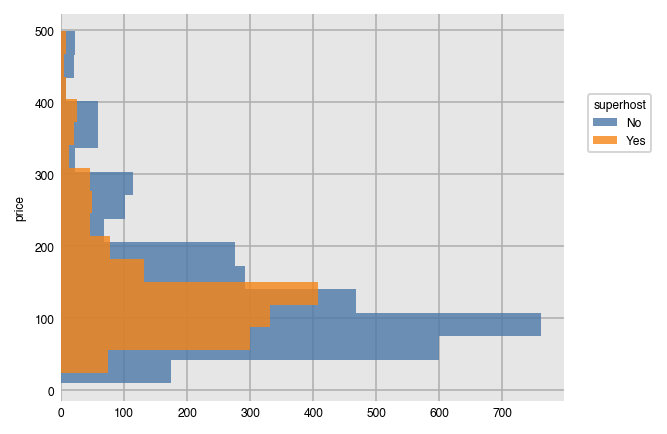Here, we customize our histogram by plotting the cumulative density as opposed to the raw frequency count using the outline of the bars ('step').

``````dxp.hist(val='price', data=airbnb, split='bedrooms', split_order=[1, 2, 3],
bins=30, density=True, histtype='step', cumulative=True)
``````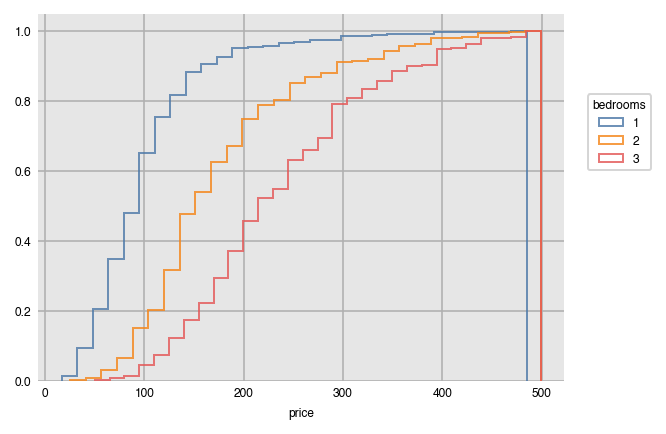### KDE Plots

Kernel density estimates provide an estimate for the probability distribution of a continuous variable. Here, we examine how price is distributed by bedroom.

``````dxp.kde(x='price', data=airbnb, split='bedrooms', split_order=[1, 2, 3])
``````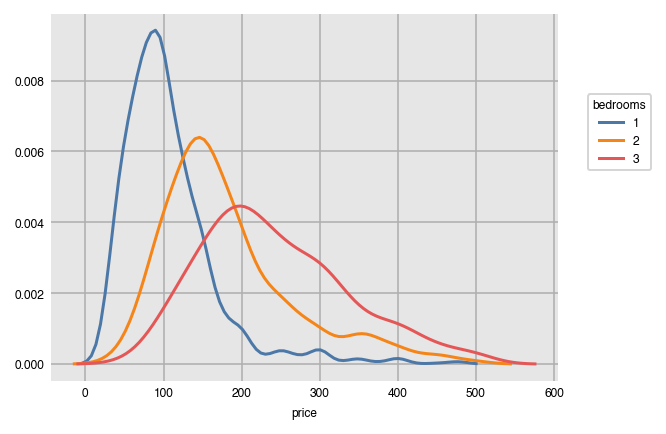Graph the cumulative distribution instead on multiple plots.

``````dxp.kde(x='price', data=airbnb, split='bedrooms',
split_order=[1, 2, 3], cumulative=True, col='property_type', wrap=2)
``````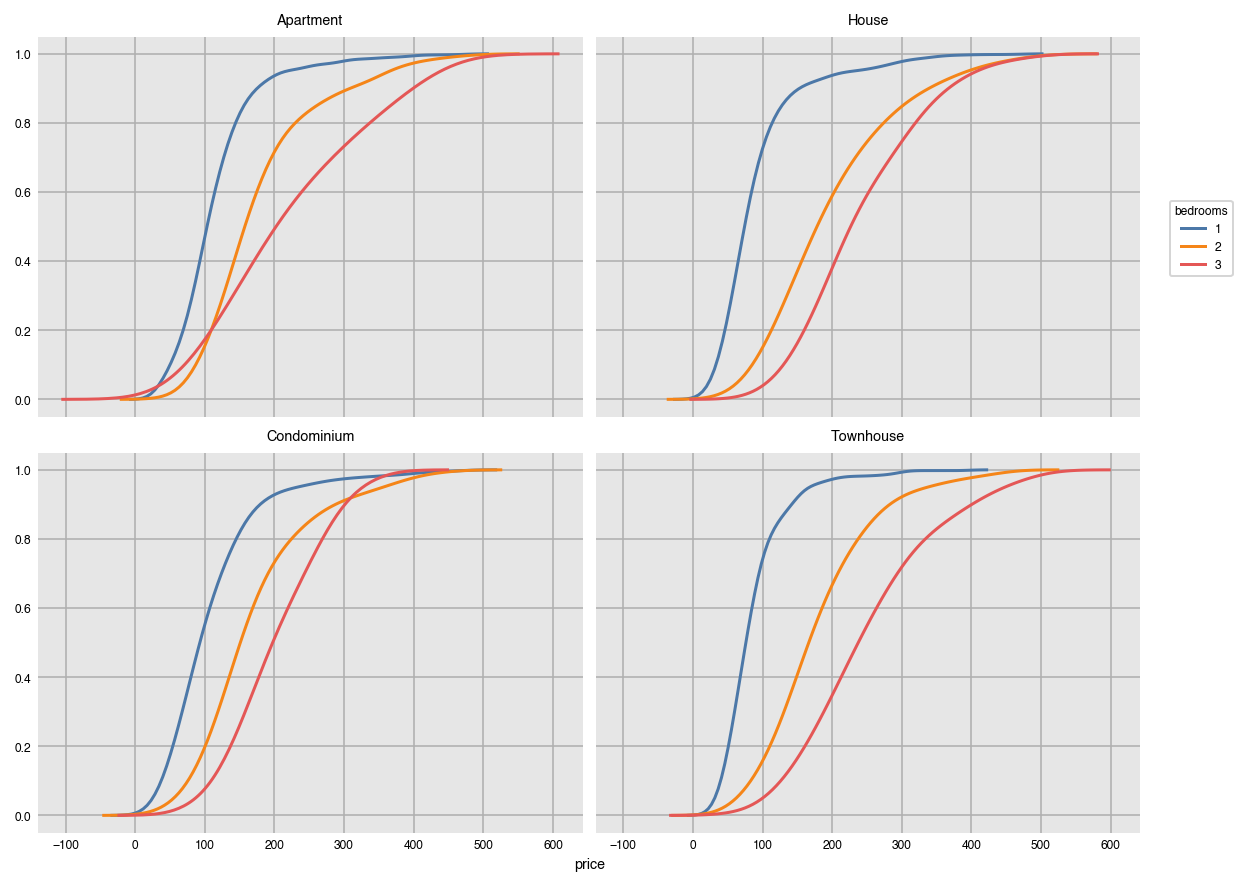### Two-dimensional KDE's

Provide two numeric columns to `x` and `y` to get a two dimensional KDE.

``````dxp.kde(x='price', y='cleaning_fee', data=airbnb)
``````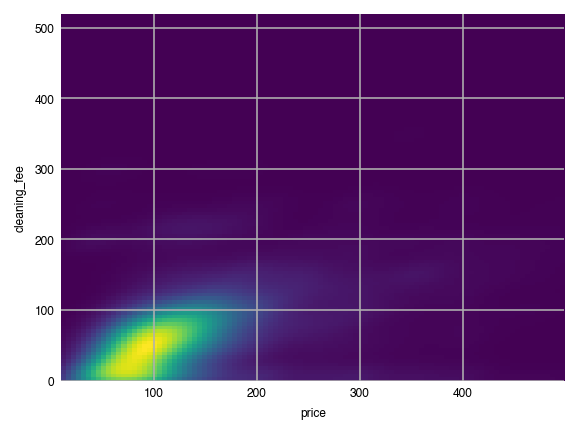Create a grid of two-dimensional KDE's.

``````dxp.kde(x='price', y='cleaning_fee', data=airbnb, row='neighborhood', wrap=3)
``````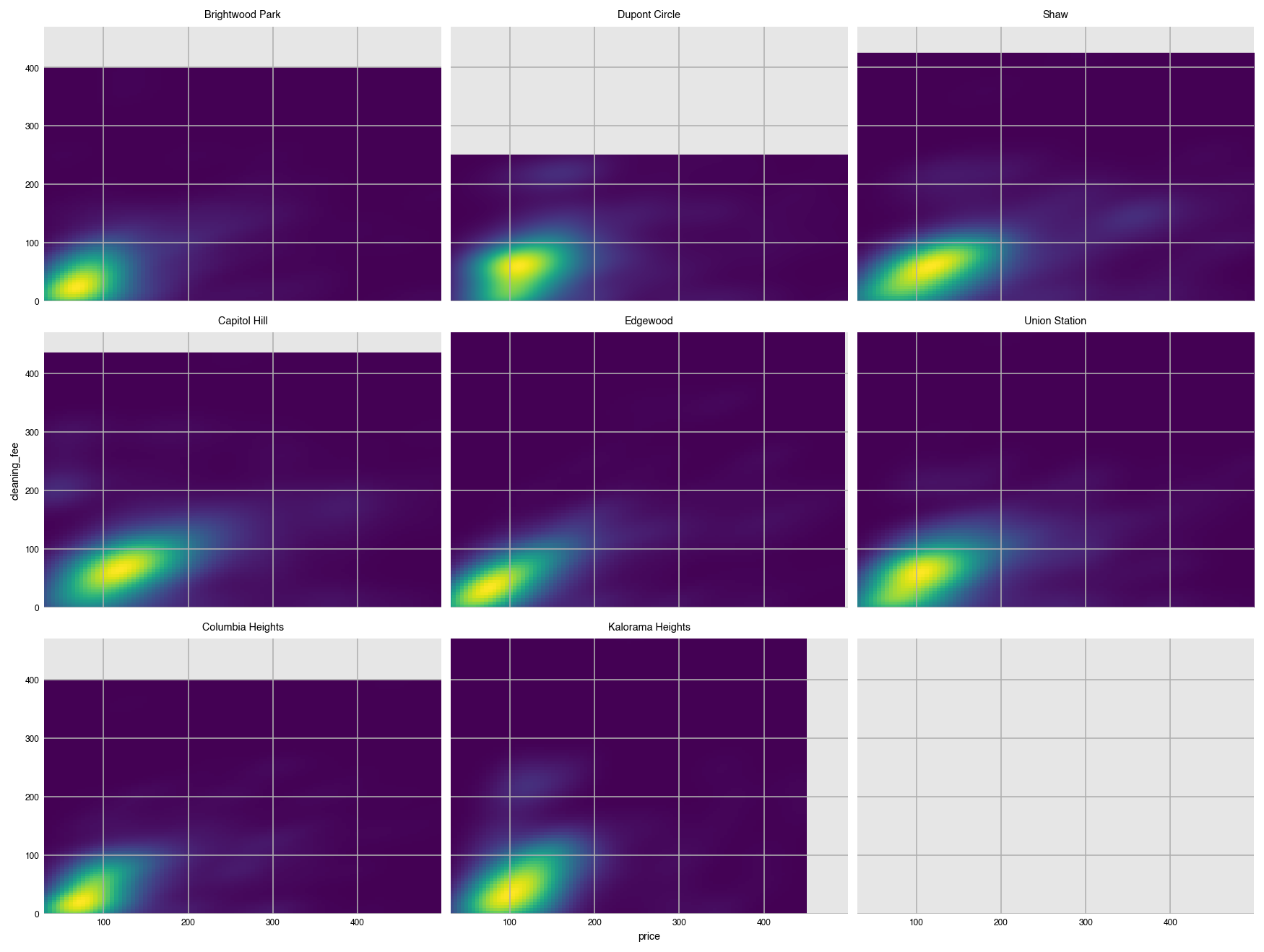## Count plots

The `count` function graphs the frequency of unique values as bars. By default, it plots the values in descending order.

``````dxp.count(val='neighborhood', data=airbnb)
``````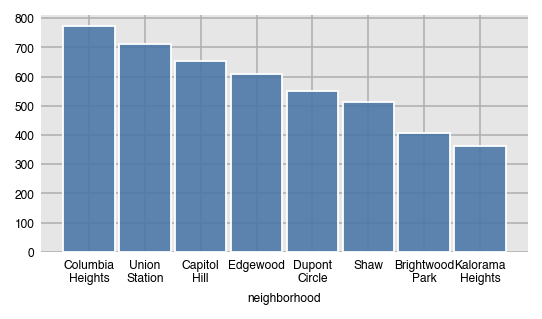In pandas, this is a straightforward call to the `value_counts` method.

``````airbnb['neighborhood'].value_counts()
``````
``````Columbia Heights    773
Union Station       713
Capitol Hill        654
Edgewood            610
Dupont Circle       549
Shaw                514
Brightwood Park     406
Kalorama Heights    362
Name: neighborhood, dtype: int64
``````

### Relative frequency with `normalize`

Instead of the raw counts, get the relative frequency by setting normalize to `True`.

``````dxp.count(val='neighborhood', data=airbnb, normalize=True)
``````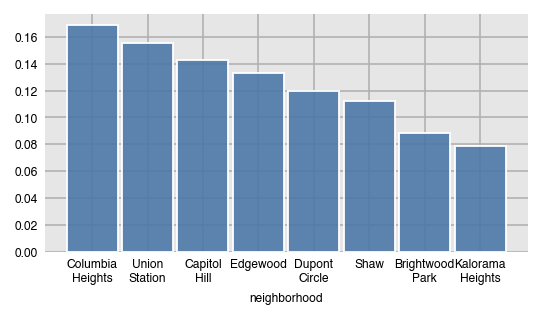Here, we split by property type.

``````dxp.count(val='neighborhood', data=airbnb, split='property_type')
``````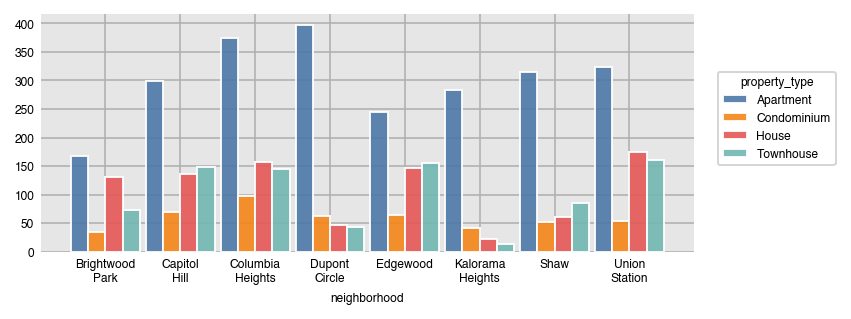In pandas, this is done with the `crosstab` function.

``````pd.crosstab(index=airbnb['property_type'], columns=airbnb['neighborhood'])
``````

Horizontal stacked count plots.

``````dxp.count(val='neighborhood', data=airbnb, split='property_type',
orientation='h', stacked=True, col='superhost')
``````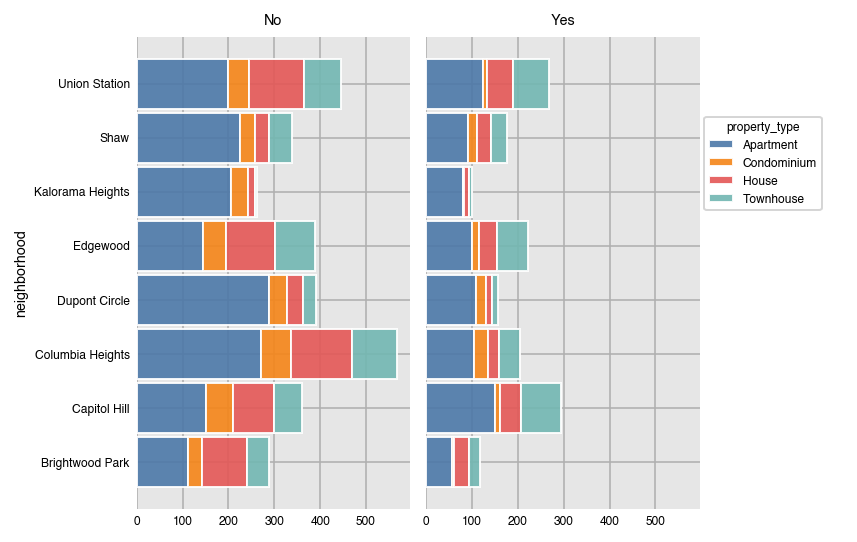### Normalize over different variables

Setting `normalize` to `True`, returns the relative frequency with respect to all of the data. You can normalize over any of the variables provided.

``````dxp.count(val='neighborhood', data=airbnb, split='property_type', normalize='neighborhood',
title='Relative Frequency by Neighborhood')
``````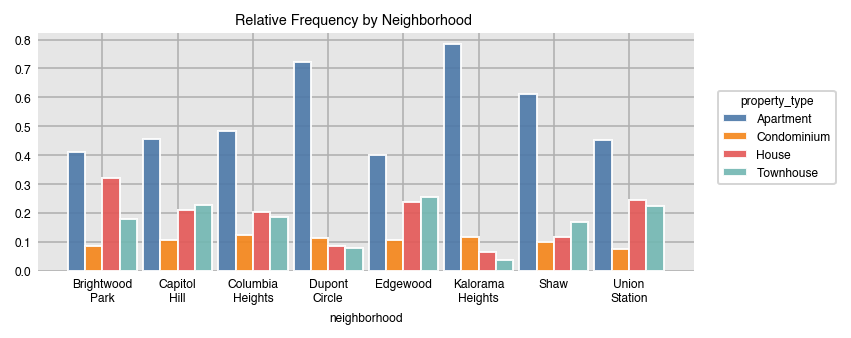Normalize over several variables at once with a list.

``````dxp.count(val='neighborhood', data=airbnb, split='superhost',
row='property_type', col='bedrooms', col_order=[1, 2],
normalize=['neighborhood', 'property_type', 'bedrooms'], stacked=True)
``````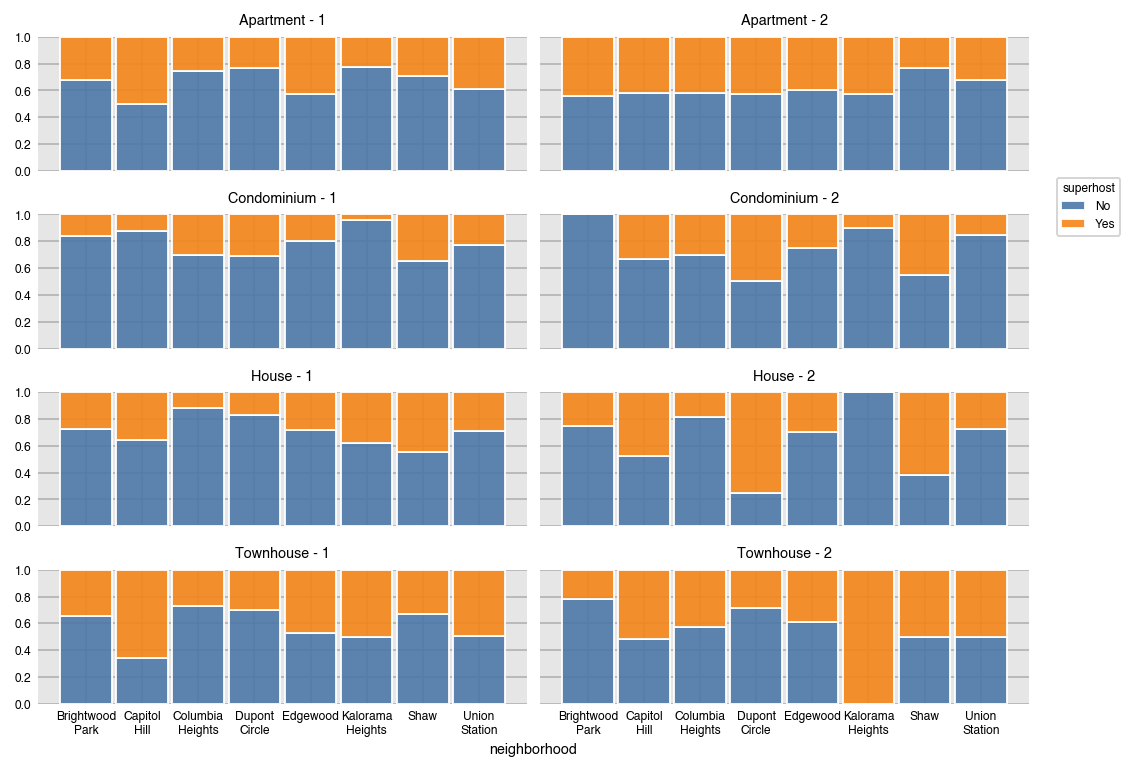## Wide data

Dexplot can also plot wide data, or data where no aggregation happens. Here is a scatter plot of the location of each listing.

``````dxp.scatter(x='longitude', y='latitude', data=airbnb,
split='neighborhood', col='bedrooms', col_order=[2, 3])
``````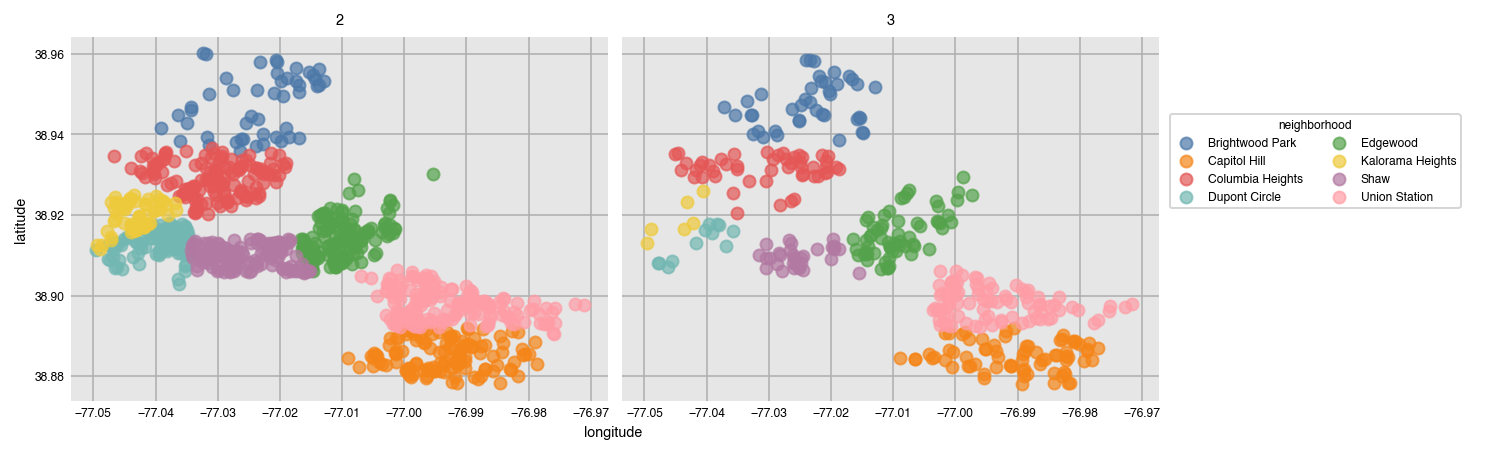If you've already aggregated your data, you can plot it directly without specifying `x` or `y`.

``````df = airbnb.pivot_table(index='neighborhood', columns='property_type',
values='price', aggfunc='mean')
df
``````
``````dxp.bar(data=df, orientation='h')
``````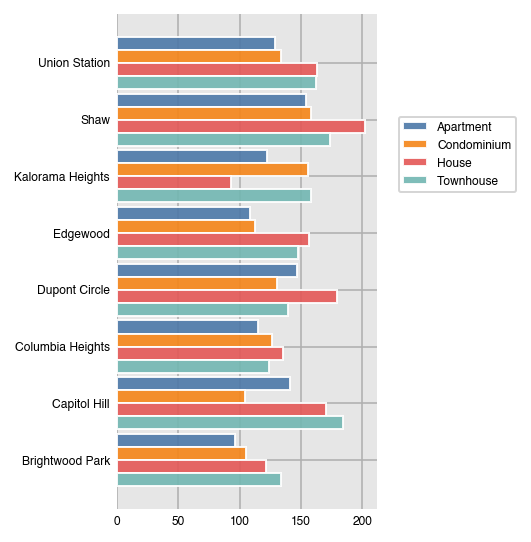### Time series

``````stocks = pd.read_csv('../data/stocks10.csv', parse_dates=['date'], index_col='date')
``````
``````dxp.line(data=stocks.head(500))
``````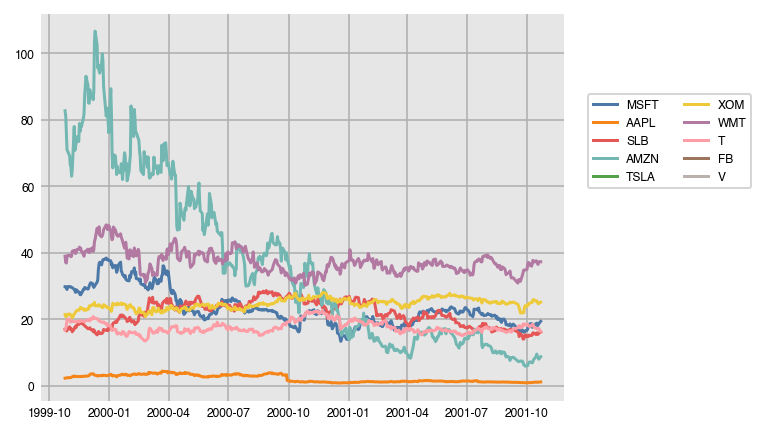Author: dexplo
Source Code: https://github.com/dexplo/dexplot

## Buddha Community1623944100

## How to Easily Show Your Matplotlib Plots and Pandas Dataframes Dynamically on Your Website

### A surprisingly easy approach to showcasing your Matplotlib plots and Pandas dataframes online for the whole world to see — in less than 100 lines of code.

Have you ever wanted to have a visualisation or dataframe accessible from your laptop or phone without having to run the code every time? Wouldn’t it be just great if you could leave it running in the background and have a web address in which you could access the data anytime, anyplace (with an internet connection)? Especially one that could automatically update when new data was available.

Turns out, you can, and it’s not that difficult at all. I taught myself how to do it over the last week just from Google, but there’s a lot of rubbish out there so here’s the easiest way that I’ve found. There might be better alternatives that I don’t know about, so please let me know if there is any. I’m genuinely curious. What you can definitely guarantee from this method, however, is simplicity. It also won’t cost you a penny. I’m also absolutely not a web developer so I apologise in advance if I murdered any terminology.

This article doesn’t expect much from you, a basic understanding of Python will do. A little knowledge of HTML would be beneficial, but absolutely not essential. Understanding Flask already would make this a piece of cake, but if you know Flask you probably know how to do this already. The structure will look a little bit like this and you could genuinely have this up and going within the hour, no problem.

• Basic routing
• Pandas Dataframes
• Matplotlib plots
• Hosting on PythonAnywhere

#data-visualization #data #python #matplotlib #pandas dataframes dynamically #how to easily show matplotlib plots and pandas dataframes dynamically on your website.

1584424980

## Matplotlib Tutorial - Bar Charts and reading in CSV Data - (Part 2)

Matplotlib Tutorial - Bar Charts and reading in CSV Data - (Part 2)
Part 2 of our Matplotlib Tutorial Videos Series is out…

In this video, we will learn creating bar charts in matplotlib. We will also learn to put bar charts side-by-side in matplotlib. Also, we will read in a csv file to create bar charts in Matplotlib .

#matplotlib #barcharts #pythonplotting #matplotlibtutorials #matplotlibvideos

#matplotlib #Matplotlib-tutorial-videos #matplotlib-plotting #matplotlib-bar-charts1623927960

## Pandas DataFrame vs. Spark DataFrame: When Parallel Computing Matters

### With Performance Comparison Analysis and Guided Example of Animated 3D Wireframe Plot

Python is famous for its vast selection of libraries and resources from the open-source community. As a Data Analyst/Engineer/Scientist, one might be familiar with popular packages such as NumpyPandasScikit-learnKeras, and TensorFlow. Together these modules help us extract value out of data and propels the field of analytics. As data continue to become larger and more complex, one other element to consider is a framework dedicated to processing Big Data, such as Apache Spark. In this article, I will demonstrate the capabilities of distributed/cluster computing and present a comparison between the Pandas DataFrame and Spark DataFrame. My hope is to provide more conviction on choosing the right implementation.

### Pandas DataFrame

Pandas has become very popular for its ease of use. It utilizes DataFrames to present data in tabular format like a spreadsheet with rows and columns. Importantly, it has very intuitive methods to perform common analytical tasks and a relatively flat learning curve. It loads all of the data into memory on a single machine (one node) for rapid execution. While the Pandas DataFrame has proven to be tremendously powerful in manipulating data, it does have its limits. With data growing at an exponentially rate, complex data processing becomes expensive to handle and causes performance degradation. These operations require parallelization and distributed computing, which the Pandas DataFrame does not support.

### Introducing Cluster/Distribution Computing and Spark DataFrame

Apache Spark is an open-source cluster computing framework. With cluster computing, data processing is distributed and performed in parallel by multiple nodes. This is recognized as the MapReduce framework because the division of labor can usually be characterized by sets of the mapshuffle, and reduce operations found in functional programming. Spark’s implementation of cluster computing is unique because processes 1) are executed in-memory and 2) build up a query plan which does not execute until necessary (known as lazy execution). Although Spark’s cluster computing framework has a broad range of utility, we only look at the Spark DataFrame for the purpose of this article. Similar to those found in Pandas, the Spark DataFrame has intuitive APIs, making it easy to implement.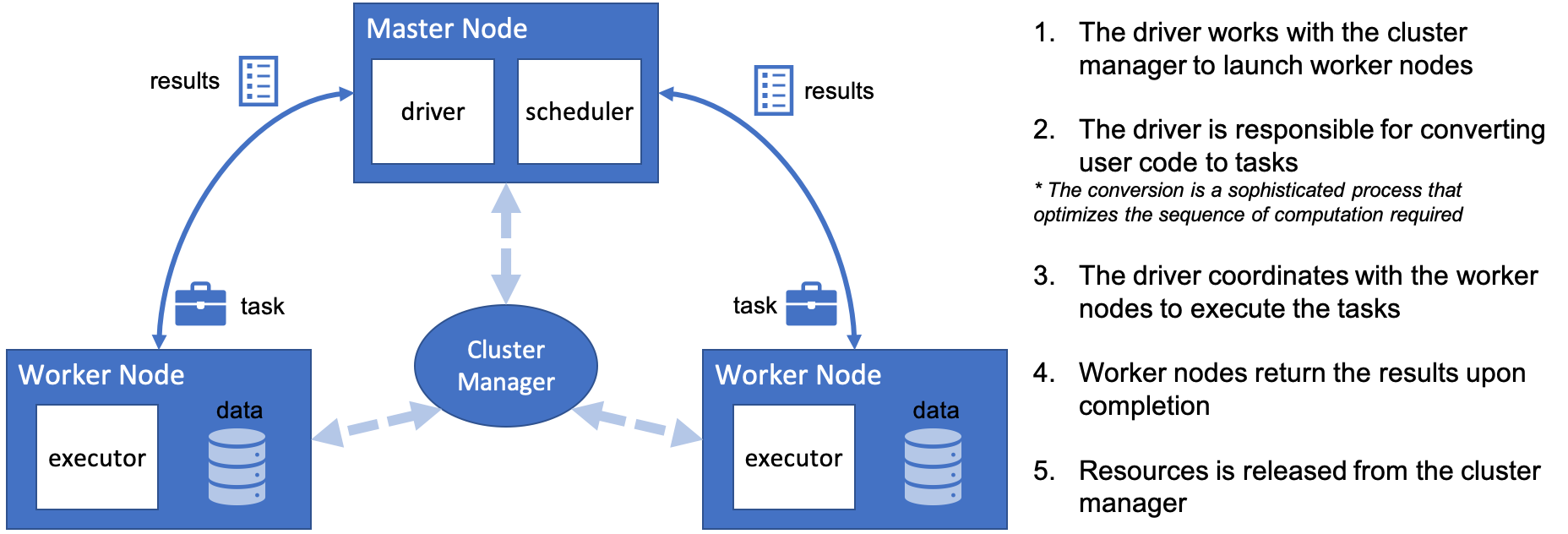#pandas dataframe vs. spark dataframe: when parallel computing matters #pandas #pandas dataframe #pandas dataframe vs. spark dataframe #spark #when parallel computing matters1592385414

## Python Matplotlib Plotting BoxPlot

This is our seventh article in Python Matplotlib, in this article we are going to learn about Matplotlib Plotting BoxPlot. so in descriptive statistics, a box plot or boxplot is a method for graphically depicting groups of numerical data through their quartiles. Box plots may also have lines extending from the boxes indicating variability outside the upper and lower quartiles, hence the terms box-and-whisker plot and box-and-whisker diagram. also Boxplot allows you to compare distributions of values by conveniently showing the median, quartiles, maximum, and minimum of a set of values.

#matplotlib #python tutorials #matplotlib boxplot #python matplotlib1592313180

## Python Matplotlib Plotting Barchart - Code Loop

This is our fourth article in Python Matplotlib, in this article we are going to learn about Matplotlib Plotting Barchart.

#matplotlib #matplotlib barchart #python #python matplotlib